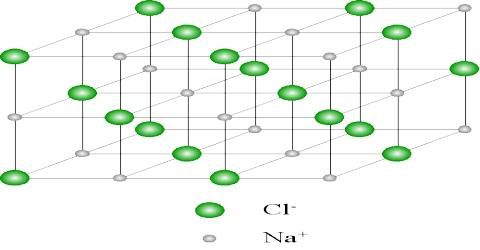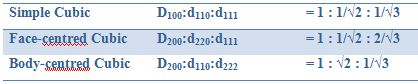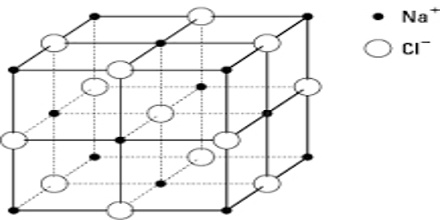Chemistry

# Structure of Sodium Chloride CrystalsThe Structure of Sodium Chloride Crystals

It is known from the external geometry of sodium chloride crystal that it belongs to the cubic system. X-ray diffraction studies with palladium target (λ = 5.81 nm ) as X-ray source shows that maxima occur from the (200), (220) and (111) faces of sodium chloride at angles 11.8°, 16.80 add 10.40 respectively (Figure: 1).Fig: Group of intensity agent 2θ for NaCl and KCl

These correspond to θ =5.9°, 8.40 and 5.20 respectively. If these are considered as first order reflections n =1, then the ratio of the spacing’s for these planes may be written from Bragg equation as:

d200 : d220 : d111 = λ/(2 Sin θ200) : λ/(2 Sin θ220) : λ/(2 Sin θ111)

= 1/(Sin θ200) : 1/(Sin θ220) : 1/(Sin θ111)

= 1/(Sin 5.9°) : 1/(Sin 8.40) : 1/(Sin 5.20)

= 1/0.1028 : 1/0.1461 : 1/0.0906

= 1 : 0.704 : 1.136 … … … (1)

It is seen in table from purely geometric consideration that for a face-centered cubic lattice.Table: Ratio of dhkl values for different structures

d200 : d220 : d111 = 1 : 1/√2 : 2/√3

= 1 : 0.707 : 1.155 … … … (2)

As values in equations (1) and (2) are in close agreement one must conclude that sodium chloride crystallizes in this lattice. The crystal lattice of sodium chloride is shown in Figure 4.6. From the difference in intensity of reflections 0f alternate orders, it was concluded that sodium chloride lattice consists of interpenetrating face-centred cubic lattice of sodium and chloride ions. In the lattice each sodium ion is surrounded by six chloride ions and vice versa (co-ordination number is six). In Figure: 2 the sodium ions are represented by small black circles and chloride ions are shown as open large circles. This is a schematic representation of the lattice. In the actual crystal the ions are probably almost touching each other so that very little free space is left. It will be noted that there are no discrete molecules of sodium chloride in the lattice.From the X-ray diffraction data the length of the unit cell may be computed. For example, for the d200 and d220 plane one obtains from the angle θ for reflection and the Bragg equation using a λ(Pd) = 5.84 nm.

d200 = 5.84 nm/2 Sin 5.90

= 5.84 nm/ 2(0.1028)

= 28.2 nm

Hence, a = d200 √(4+0+0) = 2 d200 = 56.4 nm.

One can readily see that the d200 and d220 planes have alternate sodium ion and chloride ions; the d111 plane consists of alternating planes containing sodium ions or chloride ions only.